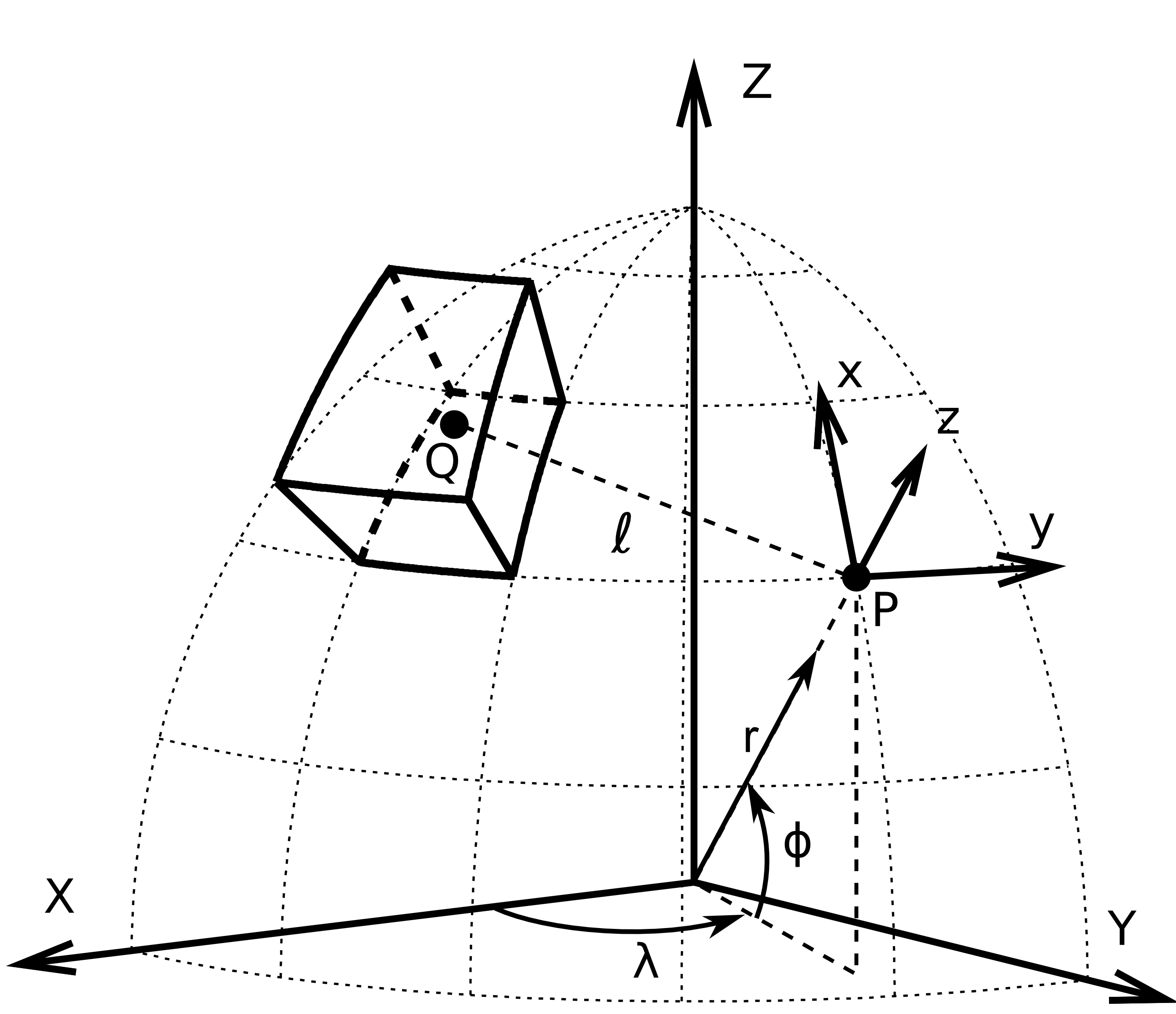The fatiando package has been deprecated. Please check out the new tools in the Fatiando a Terra website: www.fatiando.org

# Forward modeling with tesseroids (fatiando.gravmag.tesseroid)¶

Forward model the gravitational fields of a tesseroid (spherical prism).

Functions in this module calculate the gravitational fields of a tesseroid with respect to the local North-oriented coordinate system of the computation point. See the figure below.A tesseroid in a geocentric coordinate system (X, Y, Z). Point P is a computation point with associated local North-oriented coordinate system (x, y, z). Image by L. Uieda (doi:10.6084/m9.figshare.1495525).

Coordinate systems

The gravitational attraction and gravity gradient tensor are calculated with respect to the local coordinate system of the computation point. This system has x -> North, y -> East, z -> up (radial direction).

Warning

The $$g_z$$ component is an exception to this. In order to conform with the regular convention of z-axis pointing toward the center of the Earth, this component only is calculated with z -> Down. This way, gravity anomalies of tesseroids with positive density are positive, not negative.

## Gravity¶

Forward modeling of gravitational fields is performed by functions:

The fields are calculated using Gauss-Legendre Quadrature integration and the adaptive discretization algorithm of Uieda et al. (2016). The accuracy of the integration is controlled by the ratio argument. Larger values cause finer discretization and more accuracy but slower computation times. The defaults values are the ones suggested in the paper and guarantee an accuracy of approximately 0.1%.

Warning

The integration error may be larger than this if the computation points are closer than 1 km of the tesseroids. This effect is more significant in the gravity gradient components.